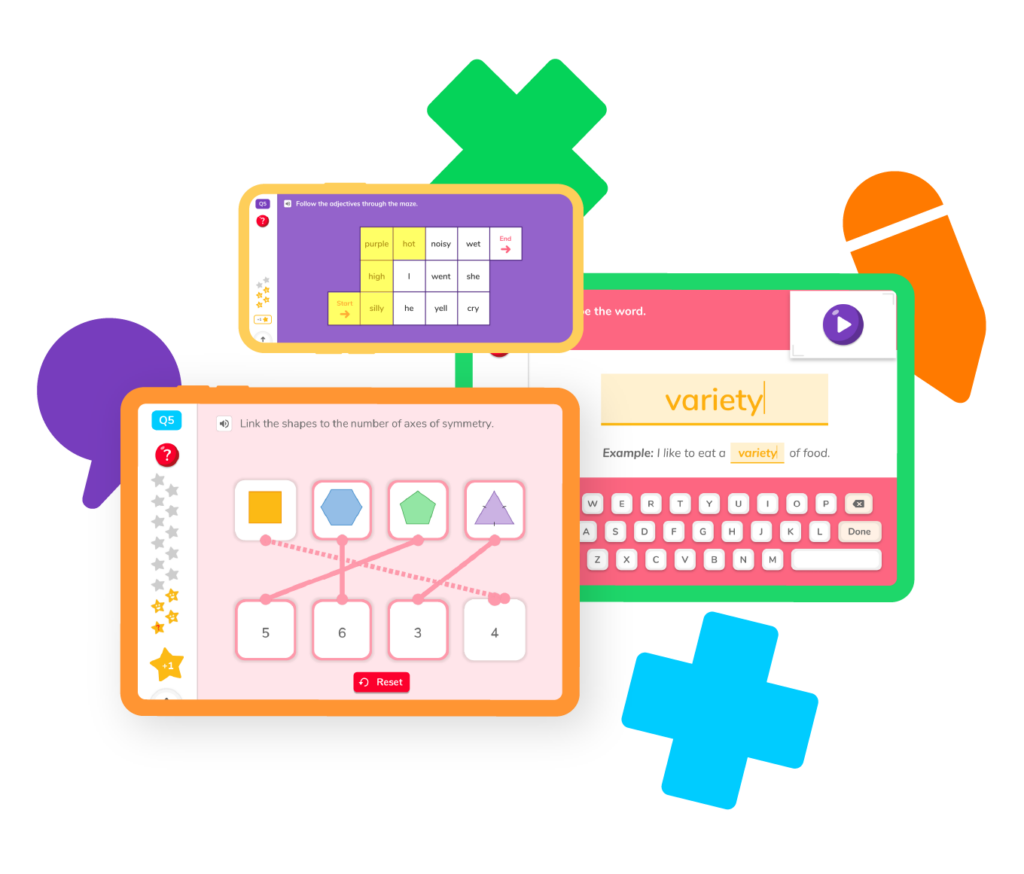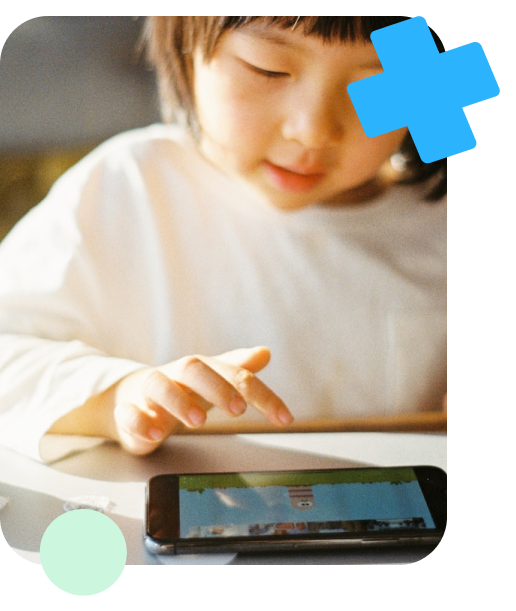They’re growing fast, and so are their math skills! DoodleMath has everything students need to succeed in 2nd grade math, from guides on place value and measurement units to exercises that will help your child grow their understanding of telling time and using money. Explore all DoodleMath has to offer and watch them master math for 2nd grade.## Select a skillFrom measuring lengths using standard units to understanding properties of operations and the relationship between addition and subtraction, 2nd graders have much to learn. Select a skill below and help your student impress their classmates and teachers with their mathematical know-how!

## Odds and evens

Recognize numbers as odd or even.

Add numbers within 20 using mental strategies.

## Shapes

Draw and recognize shapes using angles, number of faces, and more.

## Measurement

Learn and use standard units to determine the length of objects.

## Place value

Use place value to add multiple two-digit numbers together at once.

## Odds and evens

Recognize numbers as odd or even.

Add numbers within 20 using mental strategies.

## Shapes

Draw and recognize shapes using angles, number of faces, and more.

## Measurement

Learn and use standard units to determine the length of objects.

## Place value

Use place value to add multiple two-digit numbers together at once.By 2nd grade, students have developed skills like counting, recognizing shapes, and adding up to the sum of 10. Now, they will build on those skills, learning to count up to 1,000, tell time to the nearest five minutes, and give the correct change when counting out money.

Let’s take a closer look at the skills your child will explore in 2nd grade math.

1. Add and subtract within 20. Students will build on their 1st grade skills, learning how to produce sums and differences within 20 using mental math.
2. Solve one- to two-step word problems within 100. Your 2nd grade student will be able to break word problems down into one or two steps and then use addition and subtraction to solve problems within 100.
3. Determine whether a group has an odd or even number of objects. Using addition and subtraction, students will determine whether a particular group has an even number or an odd number of objects.

### Place value

1. Count to 1,000. Using their understanding of 1s, 10s, and 100s, students will learn how to count to 1,000. This includes how to skip-count using 5s, 10s, and 100s.
2. Understand that a three-digit number includes 1s, 10s, and 100s. For example, students will understand that the number 306 consists of three 100s, zero 10s, and six 1s.
3. Use place value to add and subtract within 1,000. Using concrete models, drawings, and strategies based on place value, students will add and subtract within 1,000.
4. Add up to four two-digit numbers using place value and properties of operations. Your child will be able to solve an equation that looks like: 42 + 35 + 26 + 18.

### Measurement

1. Use rulers, yardsticks, measuring tapes, and meter sticks to measure length. Students will be able to use standard units of measurement to communicate the length of objects.
2. Measure the length of an object with two different units of measurement. For example, 2nd graders will be able to tell you that 12 inches is equal to a little over 30 centimeters.
3. Measure in inches, feet, centimeters, and meters. These units of measurement will come in handy as they begin to interact with the world around them.
4. Work with time. Your 2nd grade student will know how to read a clock and tell time to the nearest 5 minutes. They can also tell you whether it is 7:05 a.m. or 7:05 p.m.
5. Work with money. Students will know the value of various coins and bills and how to exchange money and produce change.

### Shapes/Geometry

1. Recognize and draw shapes using specific attributes. If a student is told that an object has four 90-degree angles and four equal sides, they will be able to tell you that the object is a square.
2. Explain how many equal-sized squares make up a rectangle. Students will be able to determine the number of squares within a larger rectangle using their measurement skills and understanding of addition.
3. Divide squares, circles, and rectangles into equal parts. They will also be able to describe these parts using words such as “halves, thirds, fourths,” etc.

Remember that all students learn at their own pace. If your student struggles with concepts they’re learning in class, you should always talk to their teacher. You can also use DoodleMath for fun, stress-free practice. Research shows that playing math games helps students learn and retain information!As always, this largely depends on your state’s standards for 2nd grade math curriculum and the teacher, but in general, 2nd grade students should be learning how to: count to 1,000, use angles and the number of sides to determine a shape, multiply and divide simple numbers, add several two-digit numbers at one time, and measure the length of objects using standard units such as centimeters, inches, etc.

There are plenty of options out there! We recommend exploring some of our math games and skill guides to help students have fun with and understand the concepts they’re learning in school. Try to chunk homework assignments and practice sessions. It’s much easier to keep students’ attention in short bursts rather than in long study sessions.

If your student doesn’t understand some of the concepts discussed in class, you may want to talk to the teacher about their performance in class. The teacher may suggest before- or after-school tutoring sessions or they may encourage you to seek out a professional tutor.

Other signs include declining performance, especially when they move from one skill to another, or increased frustration with certain skills or concepts. Asking the teacher for extra help or hiring a math tutor — maybe even a skilled high school student — is a great way to help build your student’s confidence.# Are you a parent, teacher or student?

Are you a parent or teacher?

## Hi there!

Book a chat with our team